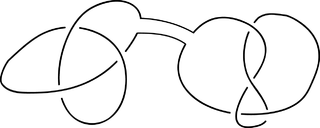# Free commutative monoid on countably many generators

Consider the following problem:

• Let$\{ n_1, n_2, \dots, n_r \}$ be a finite set of positive integers. Suppose we colour the positive integers with k distinct colours. Prove that there exist constants a and b such that$\{ a n_1^b, a n_2^b, \dots, a n_r^b\}$ is monochromatic.

Initially, this seems as though it should be a pretty difficult problem. After all, the equations are not linear due to the scary exponent b, so we can’t (for example) appeal to Rado’s theorem. However, closer inspection will reveal that at no point are we using the additive structure of the ring of integers, but instead just the multiplicative structure.

And the multiplicative structure of the positive integers is a very simple and ubiquitous object, thanks to the Fundamental Theorem of Arithmetic. In fact, it is rather surprising that its most succinct name is the free commutative monoid on countably many generators. To see this isomorphism, consider the prime factorisation of the integer:

•$\theta(n) = \theta(2^{a_1} 3^{a_2} 5^{a_3} 7^{a_4} \dots) = (a_1, a_2, a_3, a_4, \dots)$.

This is a bijection between the positive integers and sequences of non-negative integers of finite support. In particular, it satisfies the delightful prosthaphaeretic property, θ(n m) = θ(n) + θ(m). If we consider the positive rationals instead of the positive integers, then this gives a group isomorphism; without the existence of inverses, however, this is just an isomorphism between monoids.

So, what happens when we pass our problem through this monoid isomorphism? We recover the statement of the Gallai-Witt theorem (multi-dimensional generalisation of van der Waerden’s theorem). Specifically, it states that if we have a finite subset S of$\mathbb{N}^d$, then we can find a monochromatic homothetic copy of S in any k-colouring of the lattice.

It is quite amusing to see how many problems on competitions such as the IMO are actually concerned with the free commutative monoid on countably many generators, and have just been pathetically disguised as number-theoretic problems concerned with divisibility. I think that Gallai-Witt is one of the few theorems that obfuscate into a reasonably concise and natural statement rather than a contrived mess under the inverse of θ.

### Knot theory

One of the main principles in knot theory is that (directed) knots under the operation of connect sum form a monoid. Here is an example of this operation:It’s not too difficult to see that when the knots are directed, this is indeed a well-defined operation. Proving that it forms a free commutative monoid is slightly more difficult, involving the following:

• Establishing that anti-knots do not exist;
• Showing that we can uniquely express a knot as a connect sum of irreducibles, up to reordering.

The first part is generally accomplished by the famous Eilenberg-Mazur swindle. As for the second, the proof proceeds by showing that ‘overlapping irreducible knots’ can be further reduced, thus establishing a proof by contradiction. As a result of this, it suffices to only tabulate irreducible (or ‘prime’) knots, as all other knots can be uniquely expressed as a connect sum of prime knots.

Taking the ‘prime’ analogy completely literally, we can (via the free commutative monoid on countably many generators) define an isomorphism φ from the naturals to the set of knots*, such that φ(a b) is the connect sum of φ(a) and φ(b). The exact choice of φ is pretty arbitrary; each φ is uniquely determined by a bijection between the set of primes and the set of prime knots.

* Strictly speaking, the set of tame knots (those with finite complexity). The set of wild knots is uncountable.

Anyway, applying φ to the problem at the beginning of this article gives a third (and much more ridiculous!) equivalent statement of Gallai-Witt:

• Assign to each distinct knot one of k colours. Suppose we have r knots,$\{N_1, \dots, N_r \}$. Prove that there exists a knot A and a positive integer b such that, when we define the knot M_i to be the connect sum of A with b copies of N_i, the knots$\{ M_1, \dots, M_r \}$ form a monochromatic set.
This entry was posted in Uncategorized. Bookmark the permalink.

### 3 Responses to Free commutative monoid on countably many generators

1.wojowu says:

A bit of a technical question – you mentioned “homothetic copy” at one point. What are homotheties in N^d?

•apgoucher says:

Oh, a scaled (by a positive integer scale factor) and translated copy of the set.